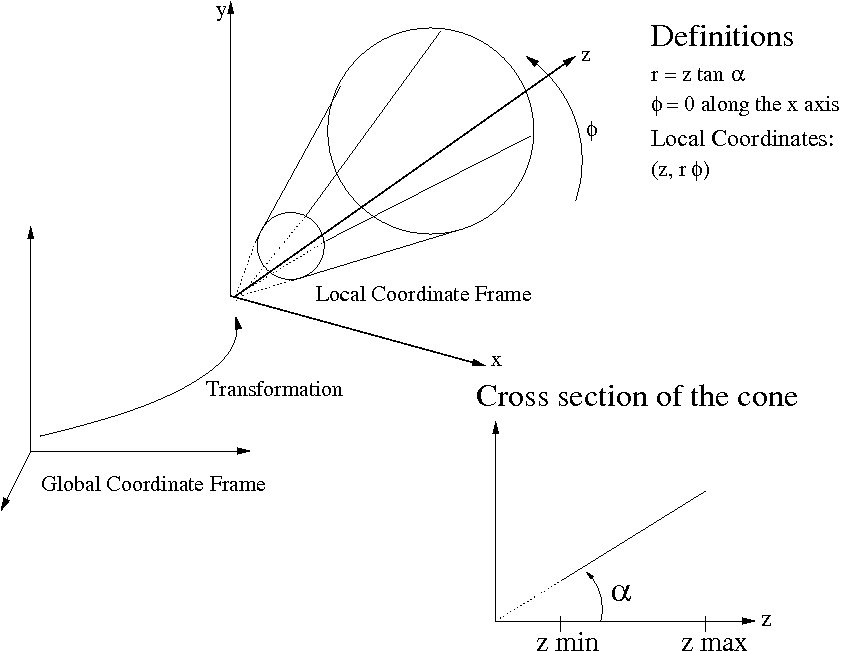# File ConeBounds.hpp

namespace Acts

Note

This file is foreseen for the Geometry module to replace Extent

class ConeBounds : public Acts::SurfaceBounds
#include <Acts/Surfaces/ConeBounds.hpp>

Bounds for a conical surface, the opening angle is stored in $$\tan(\alpha)$$ and always positively defined.

The cone can open to both sides, steered by $$z_min$$ and $$z_max$$.Public Types

enum BoundValues

Values:

enumerator eAlpha
enumerator eMinZ
enumerator eMaxZ
enumerator eHalfPhiSector
enumerator eAveragePhi
enumerator eSize

Public Functions

ConeBounds() = delete
ConeBounds(const std::array<double, eSize> &values) noexcept(false)

Constructor - from parameters array.

Parameters

values – The parameter array

ConeBounds(double alpha, bool symm, double halfphi = M_PI, double avphi = 0.) noexcept(false)

Constructor - open cone with alpha, by default a full cone but optionally can make a conical section.

Parameters
• alpha – is the opening angle of the cone

• symm – is the boolean indicating if the cone is symmetric in +/- z

• halfphi – is the half opening angle (default is pi)

• avphi – is the phi value around which the bounds are opened (default=0)

ConeBounds(double alpha, double minz, double maxz, double halfphi = M_PI, double avphi = 0.) noexcept(false)

Constructor - open cone with alpha, minz and maxz, by default a full cone but can optionally make it a conical section.

Parameters
• alpha – is the opening angle of the cone

• minz – cone expanding from minimal z

• maxz – cone expanding to maximal z

• halfphi – is the half opening angle (default is pi)

• avphi – is the phi value around which the bounds are opened (default=0)

~ConeBounds() override = default
inline double get(BoundValues bValue) const

Parameters

bValue – the class nested enum for the array access

virtual bool inside(const Vector2 &lposition, const BoundaryCheck &bcheck = true) const final

inside method for local position

Parameters
• lposition – is the local position to be checked

• bcheck – is the boundary check directive

Returns

is a boolean indicating if the position is inside

inline double r(double z) const

Return the radius at a specific z values.

Parameters

z – is the z value for which r is requested

Returns

is the r value associated with z

inline double tanAlpha() const

Return tangent of alpha (pre-computed)

virtual std::ostream &toStream(std::ostream &sl) const final

Output Method for std::ostream.

Parameters

sl – is the ostrea into which the dump is done

Returns

is the input object

virtual BoundsType type() const final

Return the bounds type - for persistency optimization.

Returns

is a BoundsType enum

inline virtual std::vector<double> values() const final

Return the bound values as dynamically sized vector.

Returns

this returns a copy of the internal values

Private Functions

inline void checkConsistency() noexcept(false)

Check the input values for consistency, will throw a logic_exception if consistency is not given.

Vector2 shifted(const Vector2 &lposition) const

Private helper function to shift a local 2D position.

Parameters

lposition – The original local position

Private Members

double m_tanAlpha
std::array<double, eSize> m_values